### Home > INT3 > Chapter Ch12 > Lesson 12.1.2 > Problem12-24

12-24.

Jeremy uses the sin–1 button on his calculator to solve a trigonometric equation and gets the solution 37º. He knows that there must be more than one solution. What are the rest of the solutions to Jeremy’s equation? Use a unit circle to explain how you determined all of the solutions. Homework Help ✎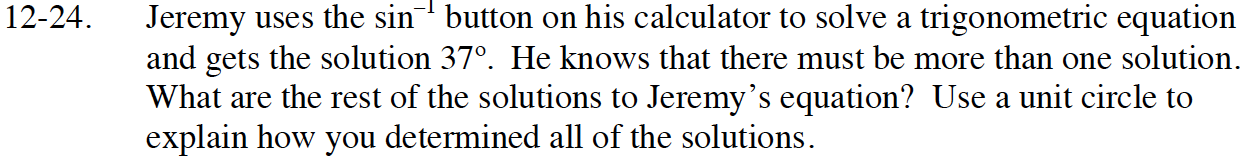Using a unit circle:

Draw a Unit circle and estimate 37 degrees.

Since sin is height, draw in the height to the x-axis.

Find and draw the same height any other place it may occur on the unit circle.

Calculate the new angles.

180° − 37° = 143°, so 37 + 2πn and 143 + 2πn where n is any integer.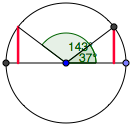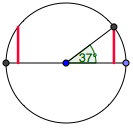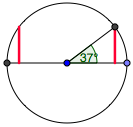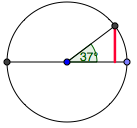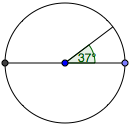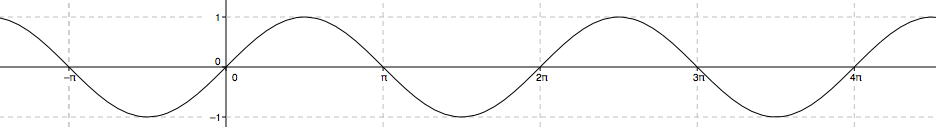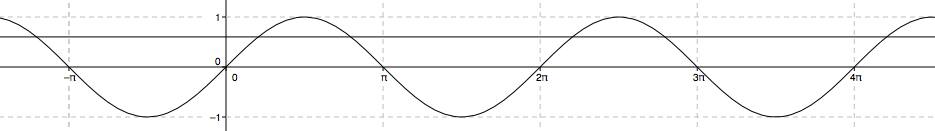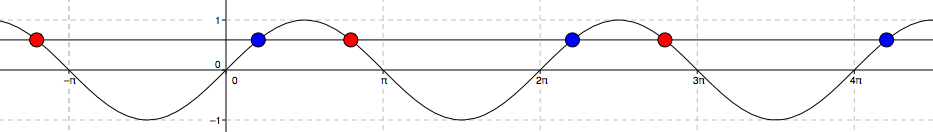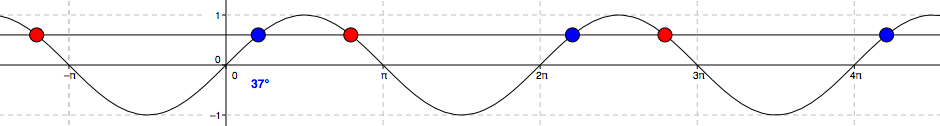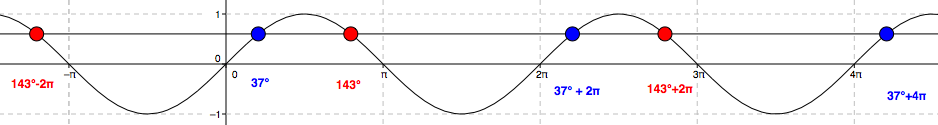Using a graph:

Draw the sine curve.

Draw y = sin(37)

Find the x-values where the two graphs intersect.

Label the solution 37°.

Use Symmetry to find other solutions.